Search by Topic

Resources tagged with Vector Notation and Geometry similar to Walls:

Filter by: Content type:
Age range:
Challenge level:

There are 20 results

Broad Topics > Vectors and Matrices > Vector Notation and GeometryVector Gem Collector

Age 14 to 18 Challenge Level:

Use vectors to collect as many gems as you can and bring them safely home!Age 16 to 18 Challenge Level:

A quadrilateral changes shape with the edge lengths constant. Show the scalar product of the diagonals is constant. If the diagonals are perpendicular in one position are they always perpendicular?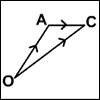An Introduction to Vectors

Age 14 to 18

The article provides a summary of the elementary ideas about vectors usually met in school mathematics, describes what vectors are and how to add, subtract and multiply them by scalars and indicates. . . .From Point to Point

Age 14 to 16 Short Challenge Level:

Can you combine vectors to get from one point to another?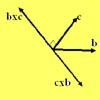Multiplication of Vectors

Age 16 to 18

An account of multiplication of vectors, both scalar products and vector products.Vector Journeys

Age 14 to 18 Challenge Level:

Charlie likes to go for walks around a square park, while Alison likes to cut across diagonally. Can you find relationships between the vectors they walk along?Areas of Parallelograms

Age 14 to 16 Challenge Level:

Can you find the area of a parallelogram defined by two vectors?Vector Racer

Age 11 to 16 Challenge Level:

The classic vector racing game.Coordinated Crystals

Age 16 to 18 Challenge Level:

Explore the lattice and vector structure of this crystal.Polygon Walk

Age 16 to 18 Challenge Level:

Go on a vector walk and determine which points on the walk are closest to the origin.Quaternions and Rotations

Age 16 to 18 Challenge Level:

Find out how the quaternion function G(v) = qvq^-1 gives a simple algebraic method for working with rotations in 3-space.Spiroflowers

Age 16 to 18 Challenge Level:

Analyse these repeating patterns. Decide on the conditions for a periodic pattern to occur and when the pattern extends to infinity.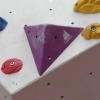Tetra Perp

Age 16 to 18 Challenge Level:

Show that the edges AD and BC of a tetrahedron ABCD are mutually perpendicular when: AB²+CD² = AC²+BD².A Knight's Journey

Age 14 to 18

This article looks at knight's moves on a chess board and introduces you to the idea of vectors and vector addition.Vector Walk

Age 14 to 18 Challenge Level:

Starting with two basic vector steps, which destinations can you reach on a vector walk?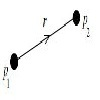Age 16 to 18 Challenge Level:

Can you arrange a set of charged particles so that none of them start to move when released from rest?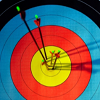Spotting the Loophole

Age 14 to 16 Challenge Level:

A visualisation problem in which you search for vectors which sum to zero from a jumble of arrows. Will your eyes be quicker than algebra?Matrix Meaning

Age 16 to 18 Challenge Level:

Explore the meaning behind the algebra and geometry of matrices with these 10 individual problems.Napoleon's Theorem

Age 14 to 18 Challenge Level:

Triangle ABC has equilateral triangles drawn on its edges. Points P, Q and R are the centres of the equilateral triangles. What can you prove about the triangle PQR?Triangle in a Triangle

Age 14 to 16 Challenge Level:

Can you work out the fraction of the original triangle that is covered by the inner triangle?